Next: Higher-Dimensional Algebra Up: Planck Previous: Topological Quantum Field Theory

# 4. 3-Dimensional Quantum Gravity

Before the late 1980s, quantum gravity was widely thought to be just as intractable in 3 spacetime dimensions as in the physically important 4-dimensional case. The situation changed drastically when physicists and mathematicians developed the tools for handling background-free quantum theories without local degrees of freedom. By now, it is easier to give a complete description of 3-dimensional quantum gravity than most quantum field theories of the traditional sort!

Let me sketch how one sets up a theory of 3-dimensional quantum gravity satisfying Atiyah's axioms for a TQFT. Before doing so I should warn reader that there are a number of inequivalent theories of 3-dimensional quantum gravity . The one I shall describe is called the Turaev-Viro model . While in some ways this is not the most physically realistic one, since it is a quantum theory of Riemannian rather than Lorentzian metrics, it illustrates the points I want to make here.

To get a TQFT satisfying Atiyah's axioms we need to describe a Hilbert space of states for each 2-dimensional manifold and an operator for each cobordism between 2-dimensional manifolds. We begin by constructing a preliminary Hilbert space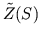for any 2-dimensional manifold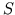. This construction requires choosing a background structure: a way of choppinginto triangles. Later we will eliminate this background-dependence and construct the Hilbert space of real physical interest.

To define the Hilbert space, it is enough to specify an orthonormal basis for it. We decree that states in this basis are ways of labelling the edges of the triangles inby numbers of the form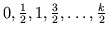. An example is shown in Figure 4, where we taketo be a sphere. Physicists call the numbers labelling the edges spins', alluding to the fact that we are using mathematics developed in the study of angular momentum. But here these numbers represent the lengths of the edges as measured in units of the Planck length. In this theory, length is a discrete rather than continuous quantity!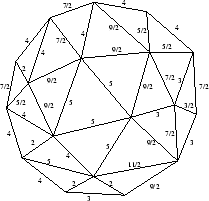Then we construct an operator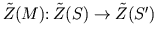for each cobordism. Again we do this with the help of a background structure on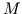: we choose a way to chop it into tetrahedra, whose triangular faces must include among them the triangles ofand. To define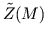it is enough to specify the transition amplitudes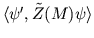whenand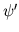are states in the bases given above. We do this as follows. The statesandtell us how to label the edges of triangles inandby spins. Consider any way to label the edges ofby spins that is compatible with these labellings of edges inand. We can think of this as a quantum geometry' for spacetime, since it tells us the shape of every tetrahedron in. Using a certain recipe we can compute a complex number for this geometry, which we think of as its amplitude' in the quantum-mechanical sense. We then sum these amplitudes over all geometries to get the total transition amplitude fromto. The reader familiar with quantum field theory may note that this construction is a discrete version of a path integral'.

Now let me describe how we erase the background-dependence from this construction. Given an identity cobordism, the operator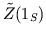is usually not the identity, thus violating one of Atiyah's axioms for a topological quantum field theory. However, the next best thing happens: this operator mapsonto a subspace, and it acts as the identity on this subspace. This subspace, which we call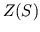, is the Hilbert space of real physical interest in 3-dimensional quantum gravity. Amazingly, this subspace doesn't depend on how we choppedinto triangles. Even better, for any cobordism, the operatormapsto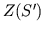. Thus it restricts to an operator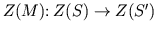. Moreover, this operatorturns out not to depend on how we choppedinto tetrahedra. To top it all off, it turns out that the Hilbert spacesand operatorssatisfy Atiyah's axioms.

In short, we started by chopping space into triangles and spacetime into tetrahedra, but at the end of the day nothing depends on this choice of background structure. It also turns out that the final theory has no local degrees of freedom: all the measurable quantities are global in character. For example, there is no operator oncorresponding to the `length of a triangle's edge', but there is an operator corresponding to the length of the shortest geodesic wrapping around the spacein a particular way. These miracles are among the main reasons for interest in quantum topology. They only happen because of the carefully chosen recipe for computing amplitudes for spacetime geometries. This recipe is the real core of the whole construction. Sadly, it is a bit too technical to describe here, so the reader will have to turn elsewhere for details [19,30]. I can say this, though: the reason this recipe works so well is that it neatly combines ideas from general relativity, quantum field theory, and a third subject that might at first seem unrelated -- higher-dimensional algebra.

Next: Higher-Dimensional Algebra Up: Planck Previous: Topological Quantum Field Theory